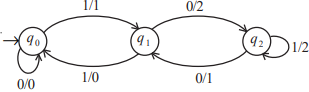# RGPV TOC June 2020

### CS-501-CBGSB.Tech., V SemesterExamination, June 2020Choice Based Grading System (CBGS)Theory of Computation

1. a) Differentiate Mealy machine and Moore machine with diagram.

b) Design Turing machine to add two number a and b.

2. a) State Pumping Lemma and show that L 1 { aibi>=1} is not a regular language.

b) Explain types of Turing machine in detail.

3. a) Prove that CFL are not closed under intersection.

b) Construct Moore machine for the following Mealy machine.4. a) Explain P class problems in detail.

b) What is a trap state in FA? Explain the properties of transition function.

Ans.

5. a) Define DFA, List three household applications of finite automata.

Ans.

b) What are leftmost and rightmost derivations? Explain with suitable example.

6. a) What is PDA? Explain instantaneous description of PDA.

b) Show that the following grammar is ambiguous.

S → aSbS|bSaS|∈

7. a) How can we construct regular grammar from regular expression

b) Write given CFG for R.E (011 + 1)*(01)*.

8. Write short note on any three of the following.

i) Undecidable problem

ii) Two way finite automata

iii) UTM

iv) Multitape

v) Recursively enumerable set

#### Read other years TOC papers.

RGPV TOC May 2018 solved paper